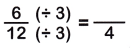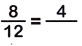# McGraw Hill Math Grade 4 Chapter 7 Lesson 3 Answer Key Finding Equivalent Fractions

Practice the questions of McGraw Hill Math Grade 4 Answer Key PDF Chapter 7 Lesson 3 Finding Equivalent Fractions to secure good marks & knowledge in the exams.

## McGraw-Hill Math Grade 4 Answer Key Chapter 7 Lesson 3 Finding Equivalent Fractions

Multiply or Divide

Multiply or divide to find equivalent fractions.

Question 1.multiplying numerator and denominator by 5
$$\frac{2}{3}$$ is equivalent to $$\frac{10}{15}$$

Question 2.Explanation:
dividing numerator and denominator by 3
$$\frac{6}{12}$$ is equivalent to $$\frac{2}{4}$$

Question 3.Explanation:
dividing numerator and denominator by 2
$$\frac{8}{12}$$ is equivalent to $$\frac{4}{6}$$

Question 4.Explanation:
multiplying numerator and denominator by 6
$$\frac{3}{4}$$ is equivalent to $$\frac{18}{24}$$

Find an equivalent fraction for each.

Question 5.
$$\frac{3}{4}$$ = ____
$$\frac{3}{4}$$ = $$\frac{9}{12}$$
Explanation:
multiplying numerator and denominator by 3

Question 6.
$$\frac{1}{3}$$ = ____
$$\frac{1}{3}$$ = $$\frac{2}{6}$$
Explanation:
multiplying numerator and denominator by 2

Question 7.
There are 30 students in a classroom. $$\frac{1}{3}$$ of their names begin with the letters M or C. How can you use equivalent fractions to find how many students this is?
$$\frac{10}{30}$$